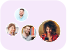Product Edition:6th Edition
Author: William M. Mendenhall, Terry L. Sincich
Book Name: Statistics for Engineering and the Sciences
Subject Name: Maths

# Statistics for Engineering and the Sciences 6th Edition Solutions

0 out of 5.0
39 reviews32 Students
have requested for homework help from this book

Prepare Your Students for Statistical Work in the Real WorldStatistics for Engineering and the Sciences Sixth Edition is designed for a two-semester introductory course on statistics for students majoring in engineering or any of the physical sciences. This popular text continues to teach students the basic concepts of data description and statistical inference as well as the statistical methods necessary for real-world applications. Students will understand how to collect and analyze data and think critically about the results.New to the Sixth EditionMany new and updated exercises based on contemporary engineering and scientific-related studies and real dataMore statistical software printouts and corresponding instructions for use that reflect the latest versions of the SAS SPSS and MINITAB softwareIntroduction of the case studies at the beginning of each chapterStreamlined material on all basic sampling concepts such as random sampling and sample survey designs which gives students an earlier introduction to key sampling issuesNew examples on comparing matched pairs versus independent samples selecting the sample size for a designed experiment and analyzing a two-factor experiment with quantitative factorsNew section on using regression residuals to check the assumptions required in a simple linear regression analysisThe first several chapters of the book identify the objectives of statistics explain how to describe data and present the basic concepts of probability. The text then introduces the two methods for making inferences about population parameters: estimation with confidence intervals and hypothesis testing. The remaining chapters extend these concepts to cover other topics useful in analyzing engineering and scientific data including the analysis of categorical data regression analysis model building analysis of variance for designed experiments nonparametric statistics statistical quality control and product and system reliability.Read more

5
20
4
8
3
5
2
1
1
5

0

## Students who viewed this book also checked out